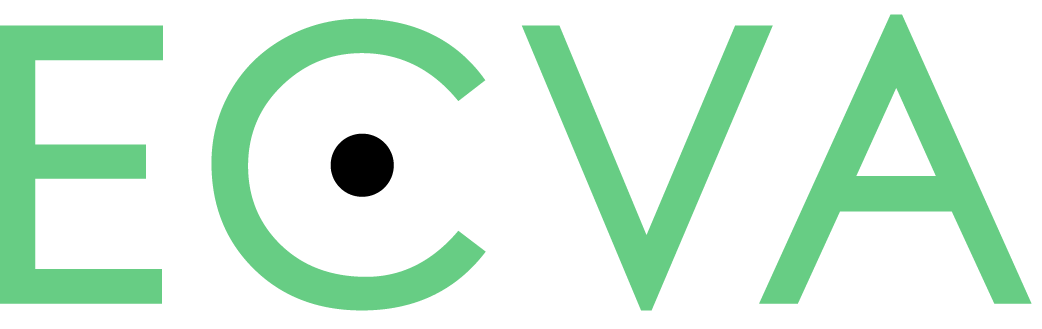#####PUGeo-Net: A Geometry-centric Network for 3D Point Cloud Upsampling

Yue Qian, Junhui Hou, Sam Kwong, Ying He ;

Abstract

In this paper, we propose a novel deep neural network based method, called PUGeo-Net, for upsampling 3D point clouds. PUGeo-Net incorporates discrete differential geometry into deep learning elegantly by learning the first and second fundamental forms that are able to fully represent the local geometry unique up to rigid motion. Specifically, we encode the first fundamental form in a 3 $imes$ 3 linear transformation matrix $\mathbf{T}$ for each input point. Such a matrix approximates the augmented Jacobian matrix of a local parameterization that encodes the intrinsic information and builds a one-to-one correspondence between the 2D parametric domain and the 3D tangent plane, so that we can lift the adaptively distributed 2D samples learned from the input to 3D space. After that, we use the learned second fundamental form to compute a normal displacement for each generated sample and project it to the curved surface. As a by-product, PUGeo-Net can compute normals for the original and generated points, which is highly desired for surface reconstruction algorithms. We evaluate PUGeo-Net on a wide range of 3D models with sharp features and rich geometric details and observe that PUGeo-Net consistently outperforms state-of-the-art methods in terms of both accuracy and efficiency for upsampling factor 4 - 16. We also verify the geometry-centric nature of PUGeo-Net quantitatively. In addition, PUGeo-Net can handle noisy and non-uniformly distributed inputs well, validating its robustness. The code is publicly available at https://github.com/ninaqy/PUGeo."

Related Material

[pdf]